# Fraction calculator

The calculator performs basic and advanced operations with fractions, expressions with fractions combined with integers, decimals, and mixed numbers. It also shows detailed step-by-step information about the fraction calculation procedure. Solve problems with two, three, or more fractions and numbers in one expression.

## Result:

### 1/3 * 2/3 = 2/9 ≅ 0.2222222

Spelled result in words is two ninths.

### How do you solve fractions step by step?

1. Multiple: 1/3 * 2/3 = 1 · 2/3 · 3 = 2/9
Multiply both numerators and denominators. Result fraction keep to lowest possible denominator GCD(2, 9) = 1. In the next intermediate step, the fraction result cannot be further simplified by canceling.
In words - one third multiplied by two thirds = two ninths.

#### Rules for expressions with fractions:

Fractions - use the slash “/” between the numerator and denominator, i.e., for five-hundredths, enter 5/100. If you are using mixed numbers, be sure to leave a single space between the whole and fraction part.
The slash separates the numerator (number above a fraction line) and denominator (number below).

Mixed numerals (mixed fractions or mixed numbers) write as non-zero integer separated by one space and fraction i.e., 1 2/3 (having the same sign). An example of a negative mixed fraction: -5 1/2.
Because slash is both signs for fraction line and division, we recommended use colon (:) as the operator of division fractions i.e., 1/2 : 3.

Decimals (decimal numbers) enter with a decimal point . and they are automatically converted to fractions - i.e. 1.45.

The colon : and slash / is the symbol of division. Can be used to divide mixed numbers 1 2/3 : 4 3/8 or can be used for write complex fractions i.e. 1/2 : 1/3.
An asterisk * or × is the symbol for multiplication.
Plus + is addition, minus sign - is subtraction and ()[] is mathematical parentheses.
The exponentiation/power symbol is ^ - for example: (7/8-4/5)^2 = (7/8-4/5)2

#### Examples:

subtracting fractions: 2/3 - 1/2
multiplying fractions: 7/8 * 3/9
dividing Fractions: 1/2 : 3/4
exponentiation of fraction: 3/5^3
fractional exponents: 16 ^ 1/2
adding fractions and mixed numbers: 8/5 + 6 2/7
dividing integer and fraction: 5 ÷ 1/2
complex fractions: 5/8 : 2 2/3
decimal to fraction: 0.625
Fraction to Decimal: 1/4
Fraction to Percent: 1/8 %
comparing fractions: 1/4 2/3
multiplying a fraction by a whole number: 6 * 3/4
square root of a fraction: sqrt(1/16)
reducing or simplifying the fraction (simplification) - dividing the numerator and denominator of a fraction by the same non-zero number - equivalent fraction: 4/22
expression with brackets: 1/3 * (1/2 - 3 3/8)
compound fraction: 3/4 of 5/7
fractions multiple: 2/3 of 3/5
divide to find the quotient: 3/5 ÷ 2/3

The calculator follows well-known rules for order of operations. The most common mnemonics for remembering this order of operations are:
PEMDAS - Parentheses, Exponents, Multiplication, Division, Addition, Subtraction.
BEDMAS - Brackets, Exponents, Division, Multiplication, Addition, Subtraction
BODMAS - Brackets, Of or Order, Division, Multiplication, Addition, Subtraction.
GEMDAS - Grouping Symbols - brackets (){}, Exponents, Multiplication, Division, Addition, Subtraction.
Be careful, always do multiplication and division before addition and subtraction. Some operators (+ and -) and (* and /) has the same priority and then must evaluate from left to right.

## Fractions in word problems:

• Coal mine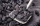The monthly plan of 17,000 tons of coal exceeded the mine by 1/25. How many tonnes of coal have been harvested from the mine above plan?
• Camp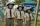In the camp are children. 1/2 went on a trip, 1/4 went to bathe, and 38 children remained in the room. How many children are in the camp?
• Multiplying fractionsWhat is 1/3 of 5?
• Reciprocals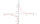Which among the given reciprocal is correct a. 3/15x1/3= 1 b. 3/20x20/3=1 c. 7/14x7/7=1 d. 34/3x34/34=1
• Tallest and shortestJenn is the tallest on the team. He is 1 1/2 times as tall as the shortest girl whom is 4 1/4 feet tall. How tall is Jebb?
• Mountain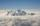Mountain has an elevation of 7450 meters and in the morning is the middle portion thereof in the clouds. How many meters of height is in the sky if below the clouds are 2,000 meters, and above clouds are two-fifths of the mountain's elevation?
• Divide 11Divide the product of 4 and 5/8 by 1 1/2. Write your answer as a mixed number.
• Length 7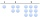Length equals 7/6 inch in width equals 7/9 inches the area is?
• Families 2Seven hundred twenty-nine families are having six children each. The probability of a girl is 1/3, and the probability of a boy is 2/3. Find the number of families having two girls and four boys.
• A triangle 2A triangle has a base of 2 inches and a height of 2 3/4 inches. What is the area of the triangle?
• A bagA bag contains 9 blue marbles and 1 green marble. What is the probability of drawing a blue marble followed by a green marble, without replacing the first marble before drawing the second marble.
• Find the 28Find the area of a square with side length 4/5 yard.
• Fraction of a fractionWhat is two-quarters of twelve?